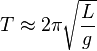# Pendulum facts for kids

Kids Encyclopedia Facts

A pendulum is a mass (any object, usually metal) on an arm. The arm is attached to a pivot, which is a point to swing from. The mass will naturally hang down because of gravity, but if it is pushed to one side it will oscillate, which means that it will swing from side to side. The frequency of the swinging pendulum stays the same.

We can work out the time taken for one full oscillation (for example swinging from left to right and back to left. We will call this time T. If the pendulum length is L, and the acceleration of gravity is g, then:$T \approx 2\pi \sqrt\frac{L}{g}\,$

Where π is read 'pi'. Note that the period of a pendulum does not depend on the mass of the object.

The mechanical energy of a pendulum is constant and is the sum of the kinetic energy and gravitational potential energy.

The regular motion of the pendulum can be used for time keeping, and pendulums are used to regulate pendulum clocks.

### 1656: The pendulum clock

In 1656 the Dutch scientist Christiaan Huygens built the first pendulum clock. This was a great improvement over existing mechanical clocks; their best accuracy was improved from around 15 minutes deviation a day to around 15 seconds a day. Pendulums spread over Europe as existing clocks were retrofitted with them.

The English scientist Robert Hooke studied the conical pendulum around 1666, consisting of a pendulum that is free to swing in two dimensions, with the bob rotating in a circle or ellipse. He used the motions of this device as a model to analyze the orbital motions of the planets. Hooke suggested to Isaac Newton in 1679 that the components of orbital motion consisted of inertial motion along a tangent direction plus an attractive motion in the radial direction. This played a part in Newton's formulation of the law of universal gravitation. Robert Hooke was also responsible for suggesting as early as 1666 that the pendulum could be used to measure the force of gravity.

During his expedition to Cayenne, French Guiana in 1671, Jean Richer found that a pendulum clock was 212 minutes per day slower at Cayenne than at Paris. From this he deduced that the force of gravity was lower at Cayenne. In 1687, Isaac Newton in Principia Mathematica showed that this was because the Earth was not a true sphere but slightly oblate (flattened at the poles) from the effect of centrifugal force due to its rotation, causing gravity to increase with latitude. Portable pendulums began to be taken on voyages to distant lands, as precision gravimeters to measure the acceleration of gravity at different points on Earth, eventually resulting in accurate models of the shape of the Earth.

## Images for kidsPendulum Facts for Kids. Kiddle Encyclopedia.